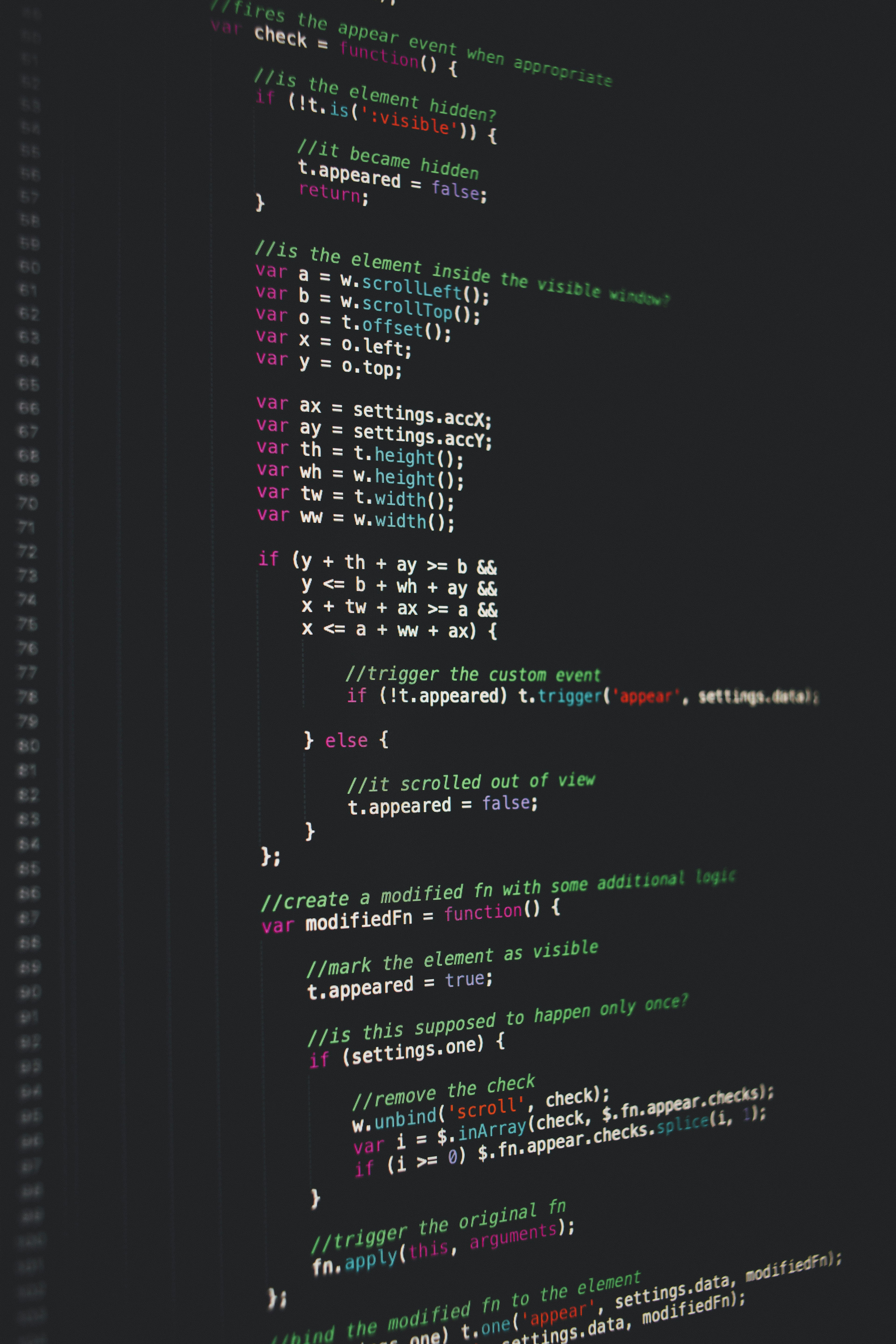Hackerrank Input and Output Solution

# Hackerrank Input and Output SolutionObjective
In this challenge, we're practicing reading input from stdin and printing output to stdout.

In C++, you can read a single whitespace-separated token of input using cin, and print output to stdout using cout. For example, let's say we declare the following variables:string s;int n;

and we want to use cin to read the input "High 5" from stdin. We can do this with the following code:cin >> s >> n;

The above code reads the first word ("High") from stdin and saves it as string , then reads the second word ("") from stdin and saves it as integer . If we want to print these values to stdout, we write the following code:cout << s << " " << n << endl;

The above code prints the contents of string , which is the word "High". Then it prints a single space (), followed by the contents of integer . Because we also want to ensure that nothing else is printed on this line, we end our line of output with a newline via endl. This results in the following output:

High 5


Read  numbers from stdin and print their sum to stdout.

Note: If you plan on completing this challenge in C instead of C++, you'll need to use format specifiers with printf and scanf.

Input Format

A single line containing  space-separated integers: , , and .

Constraints

Output Format

Print the sum of the three numbers on a single line.

Sample Input

1 2 7


Sample Output

10


Explanation

The sum of the three numbers is .

### Solution in cpp

Approach 1.


#include <cmath>
#include <cstdio>
#include <vector>
#include <iostream>
#include <algorithm>
using namespace std;

int main() {
int x,y,z;
cin>>x>>y>>z;
cout<<x+y+z;
return 0;
}


Approach 2.

#include <cmath>
#include <cstdio>
#include <vector>
#include <iostream>
#include <algorithm>
using namespace std;

int main() {
int a,b,c,sum=0;
cin>>a>>b>>c;
sum=a+b+c;
cout<<sum;
return 0;
}



Approach 3.

#include <cmath>
#include <cstdio>
#include <vector>
#include <iostream>
#include <algorithm>
using namespace std;

int main() {
int a,b,c;
cin>>a>>b>>c;
int sum=a+b+c;
cout<<sum <<endl;
return 0;
}